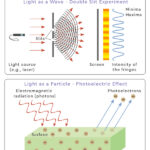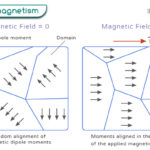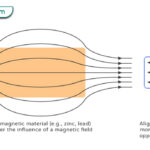Home / Physics / Magnetic Permeability

# Magnetic Permeability

Magnetic permeability refers to a material’s ability to allow the passage of magnetic field lines. It is a fundamental property that helps determine the magnetic behavior of different materials and quantifies how easily they can be magnetized in the presence of an external magnetic field. Materials with high permeability are considered highly responsive to magnetic fields. In contrast, those with low permeability offer more resistance to magnetization.

## Formula

Magnetic permeability is typically denoted by the Greek symbol μ (mu) and is given by

$\mu = \frac{B}{H}$

B represents the magnetic field strength, and H represents the magnetic field intensity.

Units

The SI unit of magnetic permeability is henry per meter (H/m) or newton per ampere squared (N/A2).

## Permeability of Free Space

The magnetic permeability of free space (μ₀) is a fundamental constant that determines how magnetic fields are formed in a vacuum. It is also known as the vacuum permeability constant. The value of μ₀ is approximately 4π × 10-7 N/A2. This constant plays a crucial role in electromagnetic theory and has various applications.

One of its most important applications is determining the speed at which electromagnetic waves propagate in free space. The speed of light in a vacuum, denoted by the symbol c, is related to μ₀ and the permittivity of free space (ε₀) through the equation

$c = \frac{1}{\sqrt{\mu_o \epsilon_o}}$

This equation shows that the speed of light depends on the properties of free space, specifically its magnetic permeability and electric permittivity. Its value is approximately 3 × 108 meters per second. Electromagnetic waves, such as light, radiowaves, and microwaves, travel at this speed when passing through a vacuum.

## Relative Permeability

The relative permeability of a material is a dimensionless quantity. It is defined as the ratio of the material’s magnetic permeability to the permeability of free space. In other words, it measures how much a material can concentrate or amplify magnetic fields compared to a vacuum.

$\mu_r = \frac{\mu}{\mu_o}$

The relative permeability of air or vacuum is considered equal to 1.

## Magnetic Permeability Values

Magnetic permeability values vary for different materials, and this property plays a crucial role in various applications involving magnets and electromagnetic systems. Here are a few relative permeability values for different materials:

Non-magnetic: Wood, plastic, glass, and most non-metallic substances have relative magnetic permeability values close to 1. They are generally considered to be non-magnetic.

Ferromagnetic: Ferromagnetic materials such as iron, nickel, and cobalt exhibit high relative magnetic permeability values, typically hundreds to thousands. This property makes them highly susceptible to becoming magnetized when exposed to a magnetic field.

Paramagnetic: Paramagnetic materials like aluminum, platinum, and oxygen have relative magnetic permeability values slightly greater than 1 but significantly less than ferromagnetic materials. They are weakly attracted to magnetic fields.

Diamagnetic: Diamagnetic materials such as copper, gold, and water have relative magnetic permeability values slightly less than 1. They are weakly repelled by magnetic fields.

It is important to note that the magnetic permeability values depend on factors such as temperature and the strength of the applied magnetic field.

Article was last reviewed on Saturday, September 30, 2023

### Related articlesWave-Particle DualityPhotoelectric EffectFerromagnetismDiamagnetism